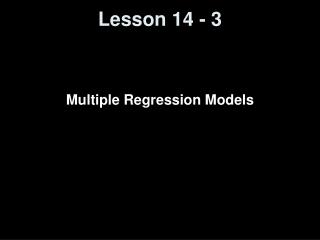DownloadDownload PresentationLesson 14 - 3

# Lesson 14 - 3

Download Presentation## Lesson 14 - 3

- - - - - - - - - - - - - - - - - - - - - - - - - - - E N D - - - - - - - - - - - - - - - - - - - - - - - - - - -
##### Presentation Transcript

1. Lesson 14 - 3 Multiple Regression Models

2. Objectives • Obtain the correlation matrix • Use technology to find a multiple regression equation • Interpret the coefficients of a multiple regression equation • Determine R2 and adjusted R2 • Perform an F-test for lack of fit • Test individual regression coefficients for significance • Construct confidence and prediction intervals • Build a regression model

3. Vocabulary • Correlation matrix – shows the linear correlation among all variables under consideration in a multiple regression model • Multicollinearity – when two explanatory variables have a high linear correlation between themselves • Additive effect – explanatory variables do not interact • Adjusted R2 – modifies the value of R2 based on the sample size, n, and the number of explanatory variables, k; will decrease if an explanatory variable is added to the model that does little to explain the variation in the response variable

4. Multiple Regression Model yi = β0 + β1x1i + β2x2i + … + βkxki + εi where yi is the value of the response variable for the ith individual β0, β1, β2, , βk ,are the parameters to be estimated based on the sample data x1iis the ithobservation for the first explanatory variable,x2iis the ithobservation for the second explanatory variable and so on εi is am independent random error term that is normally distributed with mean 0 and variance = σ² i = 1, 2, 3, …, n, where n is the sample size Note: although formulas exists to estimate β0, β1, β2, … , βkexist, we will use Excel to obtain estimates

5. Correlation Matrix • Its good that explanatory variables are highly correlated (either positively or negatively) with the response variable • There may be problems if the explanatory variables are highly correlated with each other (multi-collinearity) • General Rule: |correlation| > 0.7  then multi-collinearity may be a problem

6. n – 1 R2adj = 1 – ------------- (1 – R2) n – k – 1 R2 and Adjusted R2 Values explained variation unexplained variation R2 = ------------------------- = 1 - ----------------------------- total variation total variation note:modifies R2 based on sample size, n, and the number of explanatory variables, k to compensate for adding more variables to the model

7. Adjusted R² • The adjusted R² is used in multiple regression models • The adjusted R² will decrease if a variable is added to the model that does little to explain the variation in the response variable. • The adjusted R² will increase if a variable is taken from the model that does little to explain the variation in the response variable.

8. Hypothesis Test in Multiple Regression • The null hypothesis is that none of the explanatory variables have a significant linear relation with the response variable • The alternative hypothesis is that at least on of the explanatory variables has a significant linear relation with the response variable

9. F Test Statistic for Multiple Regression Mean Square due to Regression MSR F = ------------------------------------------- = ------------ Mean Square Error MSE F – Test Statistic Using R2 R2 n – (k + 1) F = ---------- · --------------- 1 – R2 k with k – 1 degrees of freedom in the numerator and, n – k degrees of freedom in the denominator where k is the number of explanatory variables n is the sample size NOTE: H0: β0 = β1 = β2 = … = βk = 0 use P-value compared to level of significance, α, for Decision Rule

10. Guidelines in Developing a Multiple Regression Model (backwards step-wise regression) • Construct a correlation matrix to help identify the explanatory variables that have a high correlation with the response variable. In addition, look for any indication that the explanatory variables are correlated with each other. If two explanatory variables have high correlation, then it’s a tip-off to watch out for multicollinearity – but not conclusive evidence. • See if the multiple regression model uses all the explanatory variables that have been identified by the researcher. • If the null hypothesis that all the slope coefficients are zero has been rejected, we proceed to look at the individual slope coefficients. Identify those slope coefficients that have small t-test statistics (hence large p-values). These are explanatory variable\ candidates that could be removed from the model. Remove one at a time and then recomputed the regression model. • Repeat Step 3 until all slope coefficients are significantly different from zero. • Use residual plots to check model appropriateness

11. Backwards Step-wise Regression • Put all possible variables into the model • Run regression model (focus on adjusted R²) • Pull out the variable with the highest p-value • one with the least likely probability of having a linear relationship with the response variable • Rerun the model • if adjusted R² goes up; repeat procedures • if adjusted R² goes down then stop

12. Example 9 on page 770 - 773

13. Summary and Homework • Summary • Given the appropriate conditions, we can perform inference on whether the slope and intercept are significantly different from 0 • We can also calculate confidence and prediction intervals to quantify the accuracy of our predictions of the response variable y • Multiple regression models are models where more than one explanatory variable is considered • Homework • pg 774 - 782: 1, 3, 4, 6, 8, 17